# 06-1350/Homework Assignment 2

Solve the following problems and submit them in class by November 2, 2006:

Question 1. Let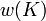$w(K)$ denote the writhe (self linking number) of a band knot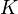$K$.

1. Is$w(K)$ a finite type invariant? Of what type?
2. In what sense is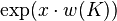$\exp(x\cdot w(K))$ "made of finite type invariants"?
3. Compute the weight system of$\exp(x\cdot w(K))$.

Question 2. Recall the HOMFLY-PT polynomial, given by the recursive definition

Failed to parse (unknown function\overcrossing): q^{N/2}H\left(\overcrossing\right)-q^{-N/2}H\left(\undercrossing\right)=(q^{1/2}-q^{-1/2})H\left(\smoothing\right)

and by the initial condition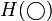$H(\bigcirc)$=1.

1. In what sense is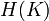$H(K)$ a finite type invariant?
2. Compute the weight system of$H(K)$.

Question 3.

1. Find a concise algorithm to compute the weight system associated with the Lie algebra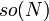$so(N)$ in its defining representation.
2. Verify that your algorithm indeed satisfies the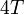$4T$ relation.

Don't submit the following, but do think about it:

Question 4. Read Dror's article Lie Algebras and the Four Color Theorem and convince yourself that it is, after all, a worthless curiosity.